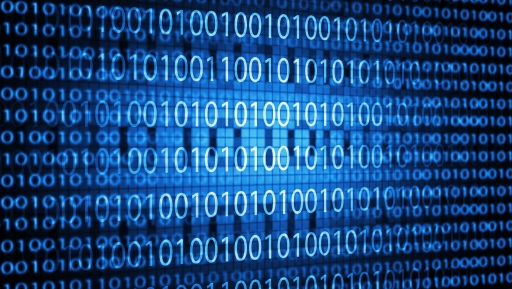Science, Maths & Technology

### Become an OU studentAn introduction to computers and computer systems

Start this free course now. Just create an account and sign in. Enrol and complete the course for a free statement of participation or digital badge if available.

# 2 Representing data: bitsIf all the data and computer instructions within a computer are represented by 1s and 0s, how can this limited set of conditions be used to represent, for instance, every letter of the alphabet that might be typed into a computer from a keyboard? Activity 1 showed that there are four possible combinations of 1s and 0s in a 2-bit binary code. If you had only two bits available you could only represent four different letters, e.g. ‘a’ could be represented by 00, ‘b’ by 01, ‘c’ by 10 and ‘d’ by 11. This shows that a 2-bit binary code can only represent four items of data.

## Activity 2

Write down all the possible combinations of a 3-bit binary code and state how many items of data three bits can represent.

To use this interactive functionality a free OU account is required. Sign in or register.
Interactive feature not available in single page view (see it in standard view).

### Discussion

The possible combinations of a 3-bit binary code are 000, 001, 010, 011, 100, 101, 110, 111. Hence the three bits can represent 8 items of data.

Interestingly, a pattern can be deduced for the relationship between the number of bits and the number of items they represent.

2 bits can represent 2 × 2 = 22 = 4 items

3 bits can represent 2 × 2 × 2 = 23 = 8 items and following on with the same pattern

4 bits can represent 2 × 2 × 2 × 2 = 24 = 16 items.

## Study note

If you are unsure of the use of the mathematical notation 22, 23 etc., you might find it helpful to refer to the following resource: Using exponent notation [Tip: hold Ctrl and click a link to open it in a new tab. (Hide tip)] .

You have seen that one binary digit of computer data is called a bit, but when we talk about computer data we often use the term byte. The next section takes you from bits to bytes.